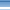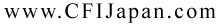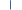HOMECONTACTLINK検索ＡＴＣ:航空無線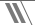筆記試験 > PVT : IFR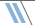ＰＴＳ:飛行試験項目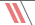お勉強室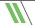航空辞書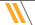けいじ板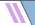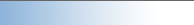# Private Pilot Ground School モドキ

D. WEIGHT AND BALANCE

1. Why do we have to calculate weight and balance? （terms)
• Weight
• Balance (CG Location)
2. Weight
• Aircraft
• Flight Crew and Passenger
• Baggage and Cargo
• Fuel
• Operating Fluids
3. Empty Weight
• Empty Weight is weight of an aircraft without people, fuel, and baggage
• Standard Empty Weight = same for same type of aircraft
• standard for each aircraft type
• unusable fuel
• full operating fluids (brake oil and others)
• full engine oil
• Basic Empty Weight
• Standard Empty Weight + Optional Equipment
• unique to each aircraft
• need to check Aircraft's Weight and Balance Data
4. Maximum Weight
• Maximum Takeoff Weight
• Maximum Landing Weight
• Maximum Ramp Weight
• Useful Load = Maximum Ramp Weight - Basic Empty Weight
5. Weight and Balance Calculation
1. Reference Point (Datum Line)
2. Weight
• Total Weight must be less than Maximum Weight
• If there is enough payload available, each person may be assumed to be 170 lbs.
• Aviation Fuel = 6 lbs / Gallon
• Engine Oil = 7.5 lbs / Gallon (if required to calculate)
3. Balance
• To find Location of CG for a safe operation
• stability
• recovery from stall and spin
• landing characteristic
• from Datum Line (each type is different)
• CG Range
• Total Moment / Total Weight = CG Location
4. Moment
• effect of distance and weight are expressed in numbers
• distance x weight
• a step to find CG Location
• effect of each item
• WEIGHT (lb) X ARM (in)= MOMENT (lb-in)
• Add all Moments then divide it by total weight
• Calculation (to find CG location)
5. Find weight and distance for all item below (for each location)
• Empty weight (from W+B Data)
• Pilot
• Passenger
• Fuel
• Oil (if required)
• Baggage and Cargo
1. Add up weight of all items to find Total Weight
2. For each item, Weight multiply by arm (distance) to find its moment.
3. Add up all the moment to calculate total Moment
4. Total Moment divide by Total Weight = CG Location
5. Check the CG location if it is within the limit
6. Use of Weight and Balance Table and Chart (Graph)